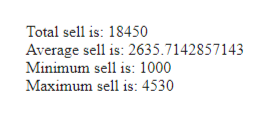# Think of one of your Math homework. How can you use program to reveal the answer? Write a calculation program with atleast ten(10) variable statements calculating a problem..Your program should include:Variables for each part of problem. See example below:Eg. \$apple = 10, \$orange = 15, \$discount = 0.2<!DOCTYPE html><html><body><?php\$apple = 10;\$orange = 15;\$discount = 0.2;echo (\$apple + \$orange) * \$discount;?></body></html>

Question
102 views

Think of one of your Math homework. How can you use program to reveal the answer? Write a calculation program with atleast ten(10) variable statements calculating a problem..

• Variables for each part of problem. See example below:

Eg. \$apple = 10, \$orange = 15, \$discount = 0.2

<!DOCTYPE html>

<html>

<body>

<?php

\$apple = 10;

\$orange = 15;

\$discount = 0.2;

echo (\$apple + \$orange) * \$discount;

?>

</body>

</html>

check_circle

Step 1

Take a math problem.

Create a program to reveal the answer to that math problem.

The program must have at least ten variables.

Step 2

Let’s take a weekly sell report and generate total sell in a week, calculate the average of the sell, find minimum sell, and find the maximum sell.

Create a PHP program to reveal the answer.

take seven variables that hold per day sell, the seven variables are:

\$monday, \$tuesday, \$wednesday, \$thursday, \$friday, \$saturday, \$sunday

Take a variable to store total sell of the week

\$totalSell

Take a variable to store average sell of the week

\$averageSell

Take a variable to store minimum sell of the week

\$minimumSell

Take a variable to store maximum sell of the week

\$maximumSell

The variable in PHP starts with a dollar(\$) sign.

Step 3

Program:

<!DOCTYPE html>

<!--html start tag-->

<html>

<!--html body start tag-->

<body>

<!--php start tag-->

<?php

/*lets take weekly sell from monday to sunday*/

\$monday = 4530;

\$tuesday = 1200;

\$wednesday = 2560;

\$thursday = 3210;

\$friday = 2530;

\$saturday = 3420;

\$sunday = 1000;

//calculate total sell of a week

\$totalSell = (\$monday + \$tuesday + \$wednesday + \$thursday + \$friday + \$saturday + \$sunday);

//calculate average sell of a week

\$averageSell = (\$totalSell/7);

//find minimum sell of a week

\$minimumSell = min(\$monday, \$tuesday, \$wednesday, \$thursday, \$friday, \$saturday, \$sunday);

//find maximum sell of a week

...help_outlineImage TranscriptioncloseTotal sell is: 18450 Average sell is: 2635.7142857143 Minimum sell is: 1000 Maximum sell is: 4530 fullscreen

### Want to see the full answer?

See Solution

#### Want to see this answer and more?

Solutions are written by subject experts who are available 24/7. Questions are typically answered within 1 hour.*

See Solution
*Response times may vary by subject and question.
Tagged in

### Computer Science